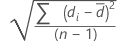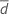# Methods and formulas for Paired t

Select the method or formula of your choice.

## Confidence interval (CI)

### Notation

TermDescription
sdΣd / n
d x1 - x2 and x1 and x2 are paired observations from populations 1 and 2, respectively
tα/2 the inverse cumulative probability of a t distribution with n-1 degrees of freedom at 1-α/2
α 1 - confidence level/100
n number of pairs of values

## T-value

### Notation

TermDescription
μ0 hypothesized population mean of the differencesmean of the paired sample differences
sd sample standard deviation of the paired sample differences
n sample size

## P-value

The calculation for the p-value depends on the alternative hypothesis.

Alternative Hypothesis P-value

### Notation

TermDescription
μd population mean for the sample of differences
μ0 hypothesized population mean for the sample of differences
tt-statistic of the sample data
tn-1a random variable from the t-distribution with n-1 degrees of freedom
nthe sample size of the differences